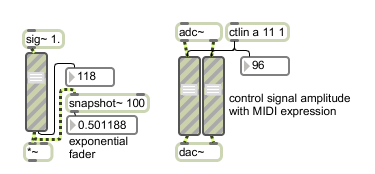gain~

Exponential scaling volume slider

Description

gain~ is a slider that scales signals. It can also make a smooth transition as you move from one value of the slider to the next. Parameters of line's formula that translates between slider values and the signal scalar can be changed via messages or by selecting the object and choosing Get Info... from the Max menu. The formula values are the same as for the linedrive object.
The default values of range (158), base value (7.94231), and increment (1.071519) provide for a slider where 128 is full scale (multiplying by 1.0), 0 produces a zero signal, and 1 is 75.6 dB below the value at 127. A change of 10 in the slider produces a 6 dB change in the output. In addition, since the range is 158, slider values from 129 to 157 provide 17.4 dB of headroom. When the slider is at 157, the output signal is 17.4 dB louder than the input signal.

None.

Messages

 bang Sends the current slider value out the right outlet. int slider-value [int] In left inlet: Sets the value of the slider, ramps the output signal to the level corresponding to the new value over the specified ramp time, and outputs the slider's value out the right outlet. float slider-value [float] In left inlet: Converted to int. (inlet1) ramp-time (milliseconds) [float] In right inlet: Sets the ramp time in milliseconds. The default is 10 milliseconds. list slider-value [list] The object takes list messages in conjunction with pattr objects. (mouse) Clicking and dragging with the mouse sets the value of the slider, ramps the output signal to the level corresponding to the new value over the specified ramp time, and outputs the slider’s value out the right outlet. set set-slider-value [int] In left inlet: The word set, followed by a number, sets the value of the slider, ramps the output signal to the level corresponding to the new value over the specified ramp time, but does not output the slider's value out the right outlet. signal In left inlet: The input signal to be scaled by the slider.

Attributes

Name Type g/s Description
bgcolor float Sets the first interior color (background) of the slider in RGBA format.
bordercolor float Sets the border color of the slider in RGBA format.
inc float
def.:1.071519
Sets the increment value used to calculate the output scale factor based on the input value. The default value is 1.071519.
interp float
def.:10.
Sets the interpolation ramp time in milliseconds for the gain~ object. The default is 10.
knobcolor float Sets the knob color of the gain~ object in RGBA format.
orientation int
def.:0
Sets the gain~ object to a horizontal or vertical data display.
0 Automatic: (the default) sets the display dependent on the size of the slider - longest side of the rectangle that describes the slider sets the orientation.
1 Horizontal
2 Vertical
relative int
def.:0
Sets way that the gain~ object responds to mouse clicks.
0 relative mode: In relative mode (the default) the gain~ keeps its relative position when you click - Moving the mouse outputs higher or lower values in relation to that relative position.
1 absolute mode: In absolute mode, the gain~ will automatically jump directly to the clicked location.
scale float
def.:7.94321
Sets the base output value used to calculate the output scale factor based on the input value. The default value is 7.94231. See the "Inspector" section for an explanation of the calculation. The word size, followed by a number, sets the range of gain~ to the number. The values of the slider will then be 0 to the range value minus 1. The default value is 158.
size int
def.:158
Sets the number of steps (range) of the gain~ object. The default value is 158.
stripecolor float Sets the second interior color (stripe) of the slider in RGBA format.

Information for box attributes common to all objects

Name Description
Color Choosing the Color... menu item from the Object menu when the object is selected opens a color picker, permitting adjustment to the appearance of the gain~ object.

Output

int: Out right outlet: The current slider value, when dragging on the slider with the mouse or when gain~ receives an int or float in its left inlet.
signal: Out left outlet: The input signal, scaled by the current slider value as x in the equation shown above.

ExamplesSpecialized fader to scale a signal exponentially or logarithmically